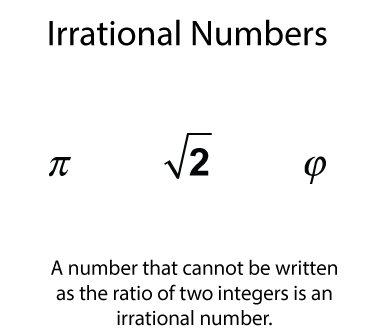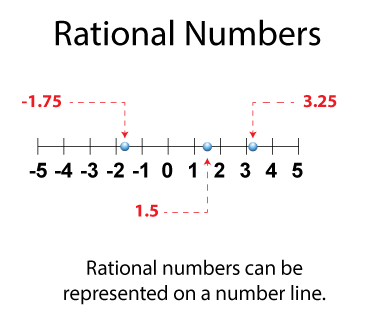# Video Tutorial: Rational Numbers: Dividing Rational Numbers

## What Are Rational Numbers?

### A Brief Review of Integers

Integers include the whole numbers, zero, and

positive numbers 1, 2, 3, etc., but also includes a different class of numbers, negative numbers.You can use a number line to represent the integers.Notice that every integer and its opposite is the same distance from 0 on the number line. Also, the arrow heads on the number line mean that the integers extend to infinity.

### Rational Numbers

All rational numbers can be written this way.Rational numbers can be written in different ways.Integers are examples of rational numbers.If a number cannot be written as the ratio of two integers, then it is an irrational number.Rational numbers can be graphed on a number line.This is part of a collection of video tutorials on the topic of Rational Numbers.

## Related Resources

To see resources related to this topic click on the Related Resources tab above.

## Math Video Library

To see the complete collection of these videos, click on this link.

Common Core Standards CCSS.MATH.CONTENT.6.RP.A.1, CCSS.MATH.CONTENT.6.NS.C.5, CCSS.MATH.CONTENT.7.NS.A.2.A, CCSS.MATH.CONTENT.8.NS.A.1, CCSS.MATH.CONTENT.6.NS.C.6, CCSS.Math.CONTENT.7.NS.A.1, CCSS.MATH.CONTENT.7.NS.A.1.C, CCSS.MATH.CONTENT.7.NS.A.1.D, CCSS.MATH.CONTENT.7.NS.A.2, CCSS.MATH.CONTENT.7.RP.A.2.C, CCSS.MATH.CONTENT.7.NS.A.2.C 4.90 minutes 6 - 8 Algebra     • Rational Expressions and Functions         • Rational Expressions 2017 rational numbers, rational numbers video tutorials, video tutorials, rational quotients, video tutorial